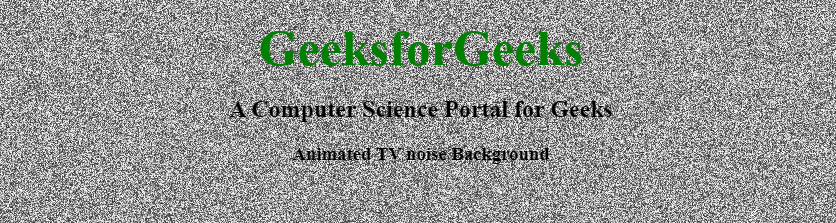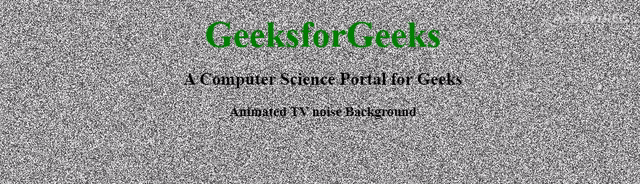# How to Create Background Like TV Noise in a Canvas ?

• Last Updated : 23 Aug, 2021TV noise is a random black and white dot pixel pattern displayed when no transmission signal is obtained by the receiver antenna of television sets or other such devices. To create that scenario as a background we will need HTML, CSS, and JavaScript. HTML is used to create the canvas area or you can use the whole background as an area. CSS will be applicable for designing the background and the JavaScript will create the TV noise animation. We will accomplish that in stepwise. The below steps are mentioned and described one by one.
HTML and CSS section:

• HTML code: Create an HTML5 canvas in webpage.

## html

 ```<``html` `lang``=``"en"``>` `<``head``>``    ``<``meta` `charset``=``"utf-8"``>``    ``<``title``>TV noise Background``` `<``body``>``    ``<``canvas` `id``=``"canvas"``>``    ``<``h1``>GeeksforGeeks``    ``<``h2``>A Computer Science Portal for Geeks``    ``<``h3``>Animated TV noise Background``` ``

• CSS code: Edit CSS of the canvas element to make it fullscreen.

## html

 `<``style``>``    ``#canvas {``        ``z-index: -100;``        ``position: absolute;``        ``top: 0;``        ``left: 0;``        ``opacity: 0.8;``        ``background-color: #fff;``    ``}``    ``h1 {``        ``color: green;``        ``font-size: 50px;``        ``margin-bottom: 0;``    ``}``    ``body {``        ``text-align: center;``    ``}```

JavaScript Section:

• JavaScript code: Write JavaScript code to create an animated TV noise effect.

## javascript

 ``

Complete solution: The following example demonstrates the creation of a background like TV noise. In this section, we just combined the HTML, CSS and JavaScript code.

## html

 ```<``html` `lang``=``"en"``>` `<``head``>``    ``<``meta` `charset``=``"utf-8"``>``    ``<``title``>TV noise Background``    ``<``style``>``        ``#canvas {``            ``z-index: -100;``            ``position: absolute;``            ``top: 0;``            ``left: 0;``            ``opacity: 0.8;``            ``background-color: #fff;``        ``}``        ` `        ``h1 {``            ``color: green;``            ``font-size: 50px;``            ``margin-bottom: 0;``        ``}``        ` `        ``body {``            ``text-align: center;``        ``}``    ````` `<``body``>``    ``<``canvas` `id``=``"canvas"``>``    ``<``h1``>GeeksforGeeks``    ``<``h2``>A Computer Science Portal for Geeks``    ``<``h3``>Animated TV noise Background` `    ``<``script``>``        ``var canvas = document.getElementById('canvas'),``            ` `        ``/* The getContext() method returns an object``        ``that provides methods and properties for``        ``drawing on the canvas. */``        ``ctx = canvas.getContext('2d');` `        ``/* Setting canvas width and height equal``        ``to window screen width and height. */``        ``function resize() {``            ``canvas.width = window.innerWidth;``            ``canvas.height = window.innerHeight;``        ``}``        ``resize();``        ``window.onresize = resize;` `        ``// Function to generate noise effect``        ``function generate_noise(ctx) {``            ``var w = ctx.canvas.width,``                ``h = ctx.canvas.height,``                ` `            ``/* This creates a new ImageData object``            ``with the specified dimensions(i.e canvas``            ``width and height). All pixels are set to``            ``transparent black (i.e rgba(0,0,0,0)). */``            ``idata = ctx.createImageData(w, h),``                ` `            ``// Creating Uint32Array typed array``            ``buffer32 = new Uint32Array(idata.data.buffer),``            ``buffer_len = buffer32.length,``            ``i = 0``            ` `            ``for (; i < ``buffer_len``; i++)``                ``buffer32[i] =``                    ``((255 * Math.random()) | 0) << 24;``            ` `            ``/* The putImageData() method puts the``            ``image data (from a specified ImageData``            ``object) back onto the canvas. */``            ``ctx.putImageData(idata, 0, 0);``        ``}` `        ``// Creating animation effect``        ``var ``toggle` `= ``true``;``        ``(function loop() {``            ``toggle = !toggle;``            ``if (toggle) {``            ` `                ``// Loop function is called each``                ``// time before next repaint``                ``requestAnimationFrame(loop);``                ``return;``            ``}``            ``generate_noise(ctx);``            ``requestAnimationFrame(loop);``        ``})();``    ````` ``

Output:My Personal Notes arrow_drop_up